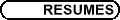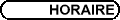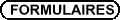SS8 - Équations différentielles et commande / SS8 - Differential Equations and Control
Org: F. Clarke (Lyon) et/and R. Stern (Concordia)

HEDY ATTOUCH, Université Montpellier II
Finite time stabilization results and dry friction

We show some finite time stabilization results concerning nonlinear oscillators subject to dry friction. Applications to physical sciences and decision sciences are given.

JEAN-PIERRE BOURGUIGNON, IHES

PIERMARCO CANNARSA, Università di Roma "Tor Vergata", Dipartimento di Matematica, Via della Ricerca Scientifica 1, 00133 Roma, Italy
Interior sphere property of attainable sets and time optimal control problems

The main object of the talk is the geometric analysis of attainable sets in time T > 0 for nonlinear control systems y¢(t) = f (y(t),u(t) ), obtaining sufficient conditions for these sets to satisfy a uniform interior sphere condition. This result is then used to recover the semiconcavity of the value function of time optimal control problems with a general target.

THIERRY CHAMPION, Université du Sud Toulon-Var
Coupling penalty schemes and the steepest descent method in convex programming

We study the asymptotic convergence of the flow associated with a dynamical system obtained by coupling the continuous steepest descent method with a penalty scheme for a convex program. The penalty parameter involved in this dynamical system may be seen as a control variable. We establish the convergence of the primal trajectories towards an optimal solution whose characterization depends on the behaviour of the penalty parameter, and we also discuss the convergence of the associates dual paths.

This is joint work with M. Courdurier (Univ. Washington, Seattle).

MONICA COJOCARU, Dept. of Mathematics, University of Guelph, Guelph, ON, Canada
Projected Differential Equations. Projected Dynamical Systems. Overview and New Developments

We present here a type of constrained dynamics, given by an ODE with discontinuous and nonlinear righthand side. Differential inclusions related to this type of equation, which we call projected differential equation (PrDE), appeared in the mathematical literature as early as 1973, then continued to be studied in the '80s. However, the definition in the form we use today was introduced in the early '90s, on Euclidean space.

Although with discontinuities, a PrDE has solutions in the class of absolutely continuous functions. We then associate a dynamics, given by these solutions, and obtain the now widely used projected dynamical system (PDS). A projected dynamics is essentially a positional control problem, since the solutions of any PrDE are constrained to evolve within and on the boundary of a closed, convex subset of the underlying space.

A crucial trait of a PrDE is that its critical points (the zeros of the righthand side) coincide with the solutions to a variational inequality problem (VI), thus making the associated projected dynamics extremely useful in applications, for example: spatial price equilibria, financial equilibria and transportation.

In this talk we present a brief history of this topic, highlighting the researchers who contributed to its advance, as well as the contribution of the author, together with collaborators, to the very recent developments in this area.

CYRIL IMBERT, Université Montpellier 2, CC 051, Place E. Bataillon, 34095 Montpellier Cedex 5
Effet régularisant d'un opérateur non local sur les équations de Hamilton-Jacobi du premier ordre

Nous étudions la régularité des solutions d'équations intégro-différentielles. Ces équations peuvent être interprétées comme des équations de Bellman-Isaacs de certains problèmes de contrôle optimal.

Les équations que nous étudions sont des équations d'Hamilton-Jacobi d'ordre 1 perturbées par un opérateur non local, le laplacien fractionnaire. Il existe déjà une littérature importante à ce sujet et la théorie des solutions de viscosité permet de donner un sens faible, non seulement aux dérivées mais aussi à l'opérateur.

Nous prouvons que sous des hypothèses naturelles sur l'Hamiltonien (celles qui assure l'unicité de la solution de l'équation non perturbée), la solution de viscosité est deux fois différentiable en espace et une fois en temps. Pour ce faire, nous construisons tout d'abord une solution de viscosité bornée et Lipschitzienne, puis nous utilisons une représentation intégrale de l'équation et des méthodes de point fixe pour prouver que la solution est en fait C2.

Nous estimons enfin la différence entre la solution de l'équation non perturbée et la solution de l'équation perturbée par un opérateur non local évanescent.

OLIVIER LEY, Université de Tours, France
Bellman-Isaacs equations under quadratic growth assumptions and applications to control

We are concerned with second-order degenerate parabolic Hamilton-Jacobi-Bellman and Isaacs equations. We prove a comparison principle between semicontinuous viscosity sub- and super-solutions growing at most quadratically.

As an application, we consider a finite horizon stochastic control problem with unbounded controls and we prove that the value function is the unique viscosity solution of the corresponding dynamic programming equation.

This is a joint work with Francesca Da Lio (Universita di Torino, Italy).

JEAN-PIERRE RAYMOND, Université Paul Sabatier, Laboratoire MIP, 31062 Toulouse Cedex 4, France
Feedback stabilization of parabolic systems

We are interested in the local stabilization of parabolic equations or systems around an unstable stationary solution. The feedback control is an internal or a boundary control. We are mainly concerned with the case where the nonlinear term of the state equation is unbounded in the space of initial data. We prove different local stabilization results by linear feedback laws (obtained by solving some algebraic Riccati equations), or by nonlinear ones (by considering Hamilton-Jacobi-Bellman equations).

Applications to the Navier-Stokes equations and to other nonlinear parabolic equations will be given.

LUDOVIC RIFFORD, Lyon

CARLO SINESTRARI, Università di Roma "Tor Vergata", Dipartimento di Matematica, Via della Ricerca Scientifica 1, 00133 Roma
Semiconcavity of the value function for exit time problems with nonsmooth target

We consider a class of nonlinear optimal control problems where the cost functional depends on the arrival time of the trajectory on a given target set. A well known example is the minimum time problem.

We study the conditions under which the value function of the problem is semiconcave. In contrast to previous works (e.g.Cannarsa-Sinestrari ) which required an interior sphere condition on the target, in the result we present here the target can be completely general. On the other hand, the dynamics of the system is assumed to satisfy suitable regularity properties. In particular, the set of admissible velocities for the system must have a smooth boundary at every point in a neighborhood of the target.

EMMANUEL TRELAT, Université Paris-Sud (Orsay), Mathématiques, Labo. AN-EDP, UMR 8628, Bat. 425, 91405 Orsay Cedex
Global subanalytic solutions of Hamilton-Jacobi type equations

In the '80s Crandall and Lions introduced the concept of viscosity solution in order to get existence and/or uniqueness results for Hamilton-Jacobi equations. We first investigate the Dirichlet and Cauchy-Dirichlet problems for such equations, where the Hamiltonian is associated to a problem of calculus of variations, and prove that if the data are analytic then the viscosity solution is moreover subanalytic. We then extend this result to Hamilton-Jacobi equations stemming from optimal control problems, in particular from sub-Riemannian geometry, which are generalized eikonal equations.

PETER WOLENSKI, Louisiana State University, Baton Rouge, Louisiana 70803
Invariance results for impulsive differential inclusions

We consider the measure-driven differential inclusion

ì
ï
í
ï
î
 dx Î F æè x(t) öø dt + G æè x(t) öø dm(t)
 x(0) = x0,
\leqno(DI)
where F : Rn \rightrightarrows Rn and G :Rn \rightrightarrows Mn×m are given multifunctions with nonempty, compact, and convex values, and m is a Borel vector-valued measure with values in a closed convex cone K Í Rm. We will discuss the nature of a "solution" to (DI) and how it can be obtained via a graphical limit of Euler-polygonal arcs. We shall also introduce and provide local characterizations of weak and strong invariance.